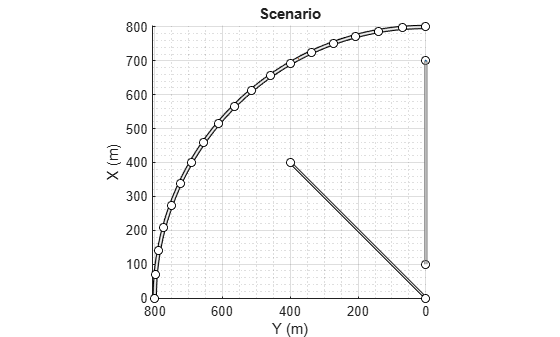# actorPoses

Positions, velocities, and orientations of actors in driving scenario

## Syntax

``poses = actorPoses(scenario)``

## Description

example

````poses = actorPoses(scenario)` returns the current poses (positions, velocities, and orientations) for all actors in the driving scenario, `scenario`. Actors include `Actor` objects, `Vehicle` objects, and `Barrier` segments, which you can create using the `actor`, `vehicle` and `barrier` functions, respectively. Actor poses are in scenario coordinates.```

## Examples

collapse all

Create a driving scenario containing a curved road, two straight roads, and two actors: a car and a bicycle. Both actors move along the road for 60 seconds.

Create the driving scenario object.

`scenario = drivingScenario('SampleTime',0.1','StopTime',60);`

Create the curved road using road center points following the arc of a circle with an 800-meter radius. The arc starts at 0°, ends at 90°, and is sampled at 5° increments.

```angs = [0:5:90]'; R = 800; roadcenters = R*[cosd(angs) sind(angs) zeros(size(angs))]; roadwidth = 10; road(scenario,roadcenters,roadwidth);```

```roadcenters = [700 0 0; 100 0 0]; road(scenario,roadcenters)```
```ans = Road with properties: Name: "" RoadID: 2 RoadCenters: [2x3 double] RoadWidth: 6 BankAngle: [2x1 double] Heading: [2x1 double] ```
```roadcenters = [400 400 0; 0 0 0]; road(scenario,roadcenters)```
```ans = Road with properties: Name: "" RoadID: 3 RoadCenters: [2x3 double] RoadWidth: 6 BankAngle: [2x1 double] Heading: [2x1 double] ```

`rbdry = roadBoundaries(scenario);`

Add a car and a bicycle to the scenario. Position the car at the beginning of the first straight road.

```car = vehicle(scenario,'ClassID',1,'Position',[700 0 0], ... 'Length',3,'Width',2,'Height',1.6);```

Position the bicycle farther down the road.

```bicycle = actor(scenario,'ClassID',3,'Position',[706 376 0]', ... 'Length',2,'Width',0.45,'Height',1.5);```

Plot the scenario.

```plot(scenario,'Centerline','on','RoadCenters','on'); title('Scenario');```Display the actor poses and profiles.

`poses = actorPoses(scenario)`
```poses=2×1 struct array with fields: ActorID Position Velocity Roll Pitch Yaw AngularVelocity ```
`profiles = actorProfiles(scenario)`
```profiles=2×1 struct array with fields: ActorID ClassID Length Width Height OriginOffset MeshVertices MeshFaces RCSPattern RCSAzimuthAngles RCSElevationAngles ```

## Input Arguments

collapse all

Driving scenario, specified as a `drivingScenario` object.

## Output Arguments

collapse all

Actor poses, in scenario coordinates, returned as a structure or an array of structures. Poses are the positions, velocities, and orientations of actors.

Each structure in `poses` has these fields.

FieldDescription
`ActorID`

Scenario-defined actor identifier, specified as a positive integer.

`Position`

Position of actor, specified as a real-valued vector of the form [x y z]. Units are in meters.

`Velocity`

Velocity (v) of actor in the x- y-, and z-directions, specified as a real-valued vector of the form [vx vy vz]. Units are in meters per second.

`Roll`

Roll angle of actor, specified as a real-valued scalar. Units are in degrees.

`Pitch`

Pitch angle of actor, specified as a real-valued scalar. Units are in degrees.

`Yaw`

Yaw angle of actor, specified as a real-valued scalar. Units are in degrees.

`AngularVelocity`

Angular velocity (ω) of actor in the x-, y-, and z-directions, specified as a real-valued vector of the form [ωx ωy ωz]. Units are in degrees per second.

For full definitions of these structure fields, see the `actor`, `vehicle` and `barrier` functions.

Introduced in R2017a